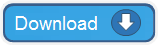# Convert Measurements

Practical application to convert a number from one measurement system to another.

It allows you to add prefixes such as giga, mega, kilo, hecto, deci, centi, milli and others to fit all your calculations.
Practical application to convert a number from one measurement system to another.

If the CONVERT function is not available on your system, install and load the Analysis ToolPak.Allows you to convert units of following measurement systems:

• Weight and Mass (gram, pound, ounce)
• Distance (meter, mile, inch, nautical mile, foot, yard, angstrom)
• Area (square meter, square mile, acre, are, square inch, square foot, square yard, square nautical mile)
• Time (year, day, hour, minute, second)
• Pressure (Pascal, atmosphere, mm of mercury)
• Force (Newton, Dina, pound force)
• Energy (Joule, Erg, calorie, electron volt, horsepower hour, watt-hour, foot pound, BTU)
• Power (horsepower, watt)
• Magnetism (Tessla, Gauss)
• Temperature (Celsius, Fahrenheit, Kelvin)
• Liquid Measure (liter, cubic meter, quart, gallon, fl. ounce, cup, spoon, pint, oil barrel)